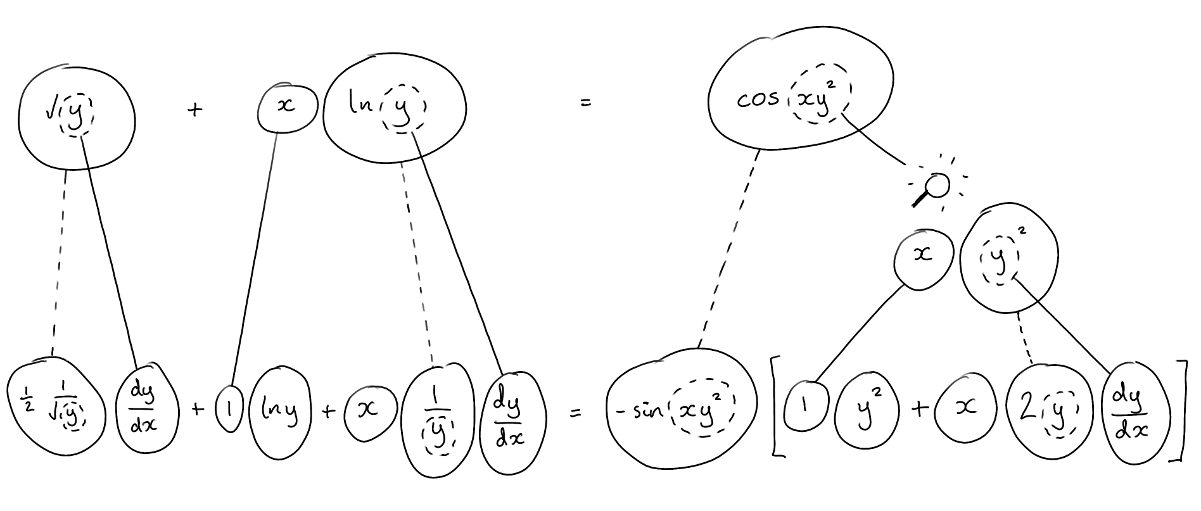# Thread: Complex numbers and Derivatives

1. ## Complex numbers and Derivatives

Hey guys, I've done more questions that I need to check.

1) a) Simplify (z-1) (z^4 + z^3 + z^2 + z + 1)

my solution: z^5 -1

b) use part a to find all the roots of the polynomial P(z) = z^4 + z^3 + z^2 + z + 1 (you may keep your answer in exponential polar form

my solution: I converted z^5 - 1 = 0 to exponential polar form and got the following roots: z = 1e^i0, 1e^i(2pi/5), 1e^i(-2pi/5), 1e^i(4pi/5), 1e^i(-4pi/5)

2) Find dy/dx if root(y) + x loge(y) = cos(xy^2)

my solution: using implicit diff and multiplication rule, i got dy/dx = [-log(y) - y^2 sin(xy^2)] / [ (x^2/2y) + 2x sin(xy^2) + (x^2/y)]

please check my solutions to these problems

thank you in advance.

2. ## Re: Complex numbers and Derivatives

Your roots are good: 5th roots of unity - Wolfram|Alpha

Simplifying the implicit differentiation is a bit messy but I'm pretty sure I don't like your (x^2)'s in the denominator.

Just in case a picture helps...... where (key in spoiler) ...

Spoiler:... is the chain rule. Straight continuous lines differentiate downwards (integrate up) with respect to the main variable (in this case x), and the straight dashed line similarly but with respect to the dashed balloon expression (the inner function of the composite which is subject to the chain rule).

This is also embedded in the legs-uncrossed version of ...... the product rule, where, again, straight continuous lines are differentiating downwards with respect to x.

Larger

I hope that helps.

__________________________________________________ __________

Don't integrate - balloontegrate!

Balloon Calculus; standard integrals, derivatives and methods

Balloon Calculus Drawing with LaTeX and Asymptote!

3. ## Re: Complex numbers and Derivatives

Hello Tom,

Thanks for the prompt reply,

I have revamped my solution fixing all silly mistakes - here it is:

dy/dx = [ -y^2sin(xy^2) - (ln(y)/2root(y)) - 1/(2root(y))] / [ x/y + 2xysin(xy^2)]

Am I correct?

Regards

4. ## Re: Complex numbers and Derivatives

No, your numerator was ok before. I suspect your differentiation is at fault.

When you differentiate the equation implicitly, do you get the bottom row of my diagram (or equivalent)? You should.

But if you do, then it's just an algebra problem. Use latex to show your steps. Click on 'Reply With Quote' and you can copy and paste the portion inside (and including) the [ tex ][ /tex ] tags, and then extend the following.

$\displaystyle \frac{1}{2} \frac{1}{\sqrt{y}} \frac{dy}{dx} + \ln y + \frac{x}{y} \frac{dy}{dx} = -\sin(xy^2) [y^2 + 2xy \frac{dy}{dx}}$

5. ## Re: Complex numbers and Derivatives

{\displaystyle \frac{1}{2} \frac{1}{\sqrt{y}} \frac{dy}{dx} + \ln y + \frac{x}{y} \frac{dy}{dx} = \sin(xy^2) [y^2 + 2xy \frac{dy}{dx}}]

Hello Balloon, I don't know how to use latex fluently, it would take me far too long to write it all out.

I will work through them in words.

First I multiplied out the brackets on the right hand side and took all the terms with a dy/dx to the left and the terms without a dy/dx to the right.
I then took out the dy/dx as a common factor and divided what was left.

6. ## Re: Complex numbers and DerivativesOriginally Posted by tom@ballooncalculusNo, your numerator was ok before. I suspect your differentiation is at fault.

When you differentiate the equation implicitly, do you get the bottom row of my diagram (or equivalent)? You should.

But if you do, then it's just an algebra problem. Use latex to show your steps. Click on 'Reply With Quote' and you can copy and paste the portion inside (and including) the [ tex ][ /tex ] tags, and then extend the following.

$\displaystyle \frac{1}{2} \frac{1}{\sqrt{y}} \frac{dy}{dx} + \ln y + \frac{x}{y} \frac{dy}{dx} = \sin(xy^2) [y^2 + 2xy \frac{dy}{dx}}$
Is that supposed to be -sin(xy^2)?

7. ## Re: Complex numbers and Derivatives

I have redone the calculations, i now get

[-y^2sin(xy^2) - loge(y)] / [ (1/(2root(y)) + (x/y) +2xy sin(xy^2)

8. ## Re: Complex numbers and DerivativesOriginally Posted by andrew2322Is that supposed to be -sin(xy^2)?
Oh, yes it is.Originally Posted by andrew2322I have redone the calculations, i now get
[-y^2sin(xy^2) - loge(y)] / [ (1/(2root(y)) + (x/y) +2xy sin(xy^2) ]
Correct!

9. ## Re: Complex numbers and Derivatives

Thanks mate, have a great night!

10. ## Re: Complex numbers and Derivatives

And you!

complex, derivatives, numbers